Function Repository Resource:

# GeometricSolve

Solve for a given quantity in a geometric scene

Contributed by: Daniel McDonald
 ResourceFunction["GeometricSolve"][scene,expr] solves for the quantity expr defined by the GeometricScene scene. ResourceFunction["GeometricSolve"][scene,{expr1,expr2,…}] returns a list containing the solutions of scene for expr1,expr2, ….

## Details and Options

Each separate solution is returned as a List.
Solution components are returned in the form expr1value1, expr2value2, ….

## Examples

### Basic Examples (3)

Solve for the hypotenuse of a right triangle:

 In:=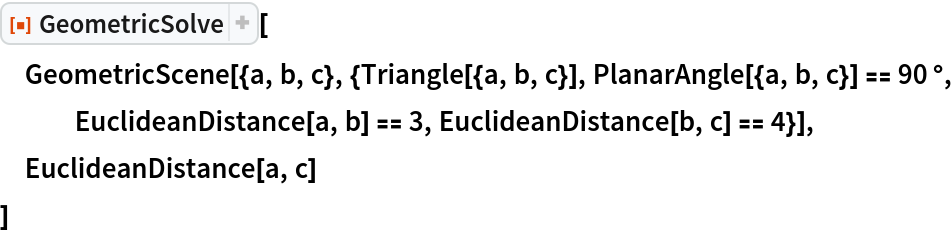Out=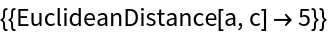Compute the area of a specific triangle:

 In:=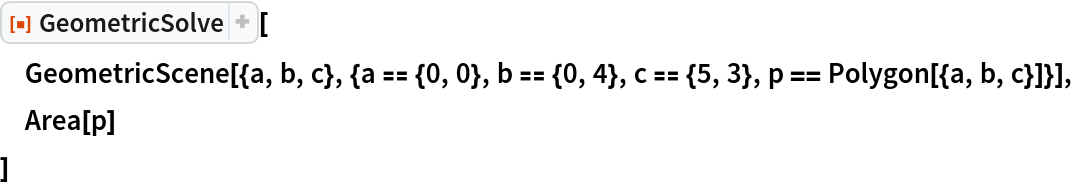Out=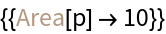Compute the area of a triangle where one point has an unknown but positive x value:

 In:=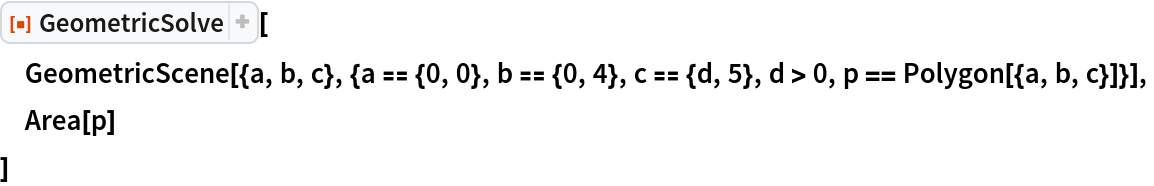Out=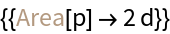### Scope (2)

For a generic triangle, there are two possible solutions for the area, only one of which will have the correct sign for a specific numeric instantiation of the parameters:

 In:=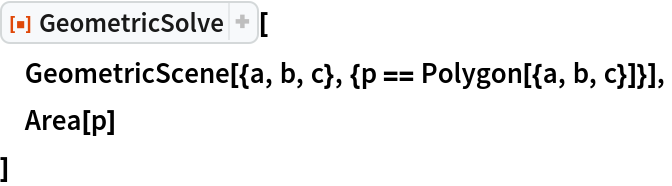Out=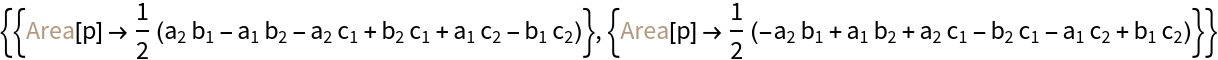Find an instance of a scene:

 In:=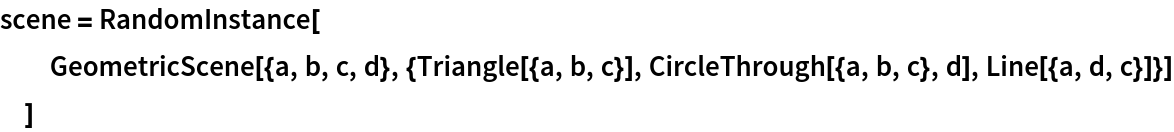Out=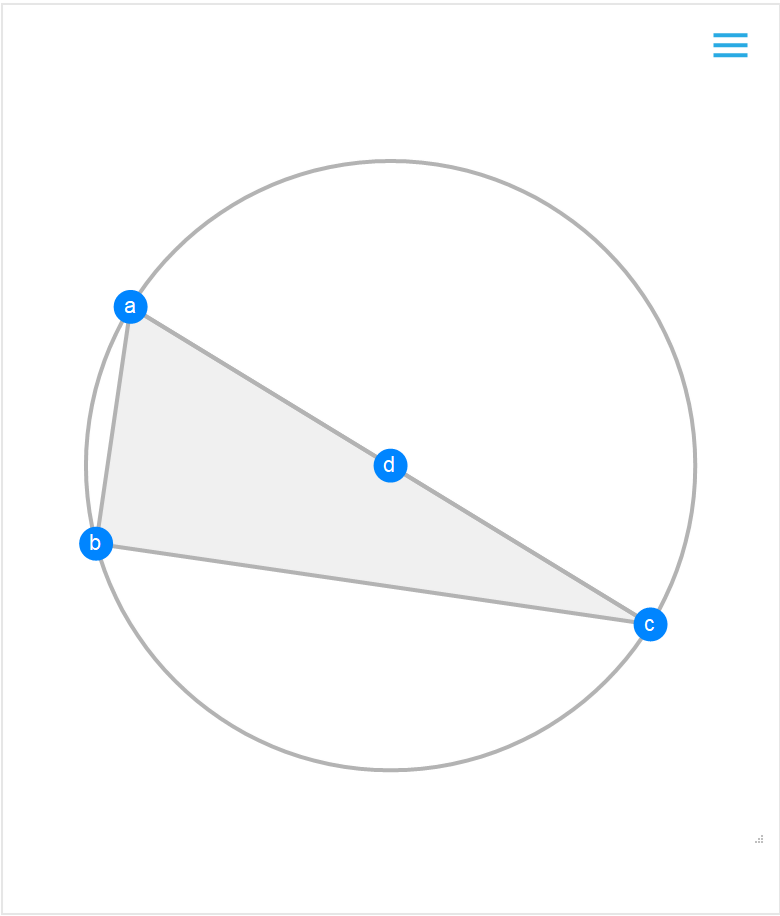Solve for the point a, the distance between points a and c and the area of the triangle in this scene instance:

 In:=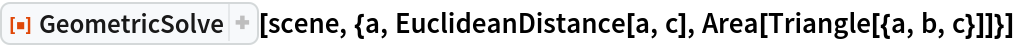Out=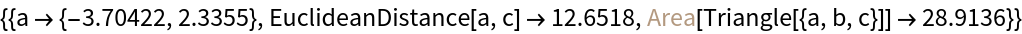Daniel McDonald

## Requirements

Wolfram Language 12.2 (December 2020) or above

## Version History

• 1.0.0 – 07 December 2020

## Author Notes

Requires Version 12.2.